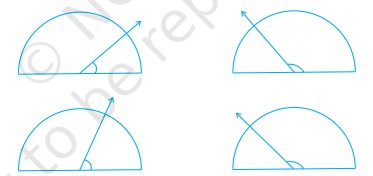" ">

# Find the measure of the angle shown in each figure. (First estimate with your eyes and then find the actual measure with a protractor)."

To do:

We have to find the measures of the angles shown in each figure.

Solution:

Estimation of the first angle: It is equal to half of the right angle.

Measuring the angle using Protractor, the first angle is equal to $40^o$

Estimation of the second angle: It is equal to three-fourths of the straight angle.

Measuring the angle using Protractor, the second angle is equal to $130^o$

Estimation of the third angle: It is equal to two-thirds of the right angle.

Measuring the angle using Protractor, the third angle is equal to $65^o$

Estimation of the fourth angle: It is equal to three-fourths of the straight angle.

Measuring the angle using Protractor, the fourth angle is equal to $135^o$

Updated on: 10-Oct-2022

14 Views